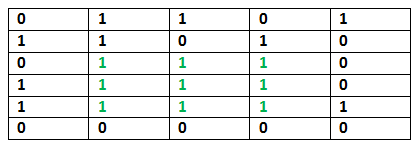# Python Program for Maximum size square sub-matrix with all 1s

Given a binary matrix, find out the maximum size square sub-matrix with all 1s.

For example, consider the below binary matrix.## Python3

 `def` `print_max_sub_matrix(grid):  ` `     `  `    ``row_len ``=` `len``(grid) ` `    ``col_len ``=` `len``(grid[``0``]) ` `     `  `    ``cache ``=` `[[``0` `for` `k ``in` `range``(col_len)] ``for` `l ``in` `range``(row_len)] ` `     `  `    ``for` `i ``in` `range``(``0``, row_len):  ` `        ``for` `j ``in` `range``(``0``, col_len): ` `            ``if` `(grid[i][j] ``=``=` `1``): ` `                ``# if row(i) or column(j) is 0, set to 1 or ` `                ``if` `i ``=``=` `0` `or` `j ``=``=` `0``: ` `                    ``cache[i][j] ``=` `min``(grid[i][j], ``1``) ` `                ``else``: ` `                    ``cache[i][j] ``=` `min``(cache[i][j``-``1``], cache[i``-``1``][j],cache[i``-``1``][j``-``1``]) ``+` `1` `            ``else``: ` `                ``cache[i][j] ``=` `0` `     `  `    ``max_in_cache ``=` `cache[``0``][``0``]  ` `    ``max_i ``=` `0` `    ``max_j ``=` `0` `     `  `    ``for` `i ``in` `range``(row_len):  ` `        ``for` `j ``in` `range``(col_len):  ` `            ``if` `max_in_cache < cache[i][j]:  ` `                ``max_in_cache ``=` `cache[i][j]  ` `                ``max_i ``=` `i  ` `                ``max_j ``=` `j  ` ` `  `    ``for` `i ``in` `range``(max_i, max_i ``-` `max_in_cache, ``-` `1``):  ` `        ``for` `j ``in` `range``(max_j, max_j ``-` `max_in_cache, ``-` `1``):  ` `            ``print` `(grid[i][j], end ``=` `" "``)  ` `        ``print``("")  ` ` `  `# Driver Program  ` `grid ``=` `[ ` `    ``[``1``, ``1``, ``1``, ``1``, ``1``],  ` `    ``[``1``, ``1``, ``1``, ``1``, ``0``],  ` `    ``[``1``, ``1``, ``0``, ``1``, ``0``],  ` `    ``[``0``, ``1``, ``1``, ``1``, ``0``],  ` `    ``[``1``, ``1``, ``0``, ``1``, ``1``],  ` `    ``[``0``, ``0``, ``0``, ``0``, ``0``] ` `]  ` ` `  `print``(``"Maximum sub-matrix:"``)  ` `print_max_sub_matrix(grid) `

Output:

```Maximum size sub-matrix is:
1 1
1 1
```

Please refer complete article on Maximum size square sub-matrix with all 1s for more details!

My Personal Notes arrow_drop_up

Improved By : chasezimmy

Article Tags :

Be the First to upvote.

Please write to us at contribute@geeksforgeeks.org to report any issue with the above content.